Puzzle Page 1 Puzzle #23       6/1/2000 Referring to the diagram below, line AB=12, Line BC=4, Line DB =3 and Line DB is perpendicular to Line AC. What is the diameter of the circle?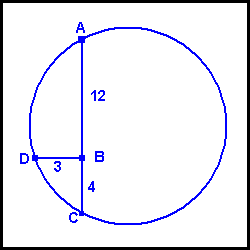ANSWER Step 1 Extend line DB out to point E. From geometry we know that when two chords of a circle intersect, the products of their segments are equal. In other words Line AB times Line BC equals Line DB times Line BE. Therefore 12 x 4 = 3 x Line BE. Line BE=16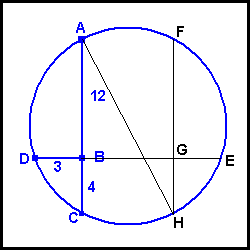Step 2 Draw Line FGH such that line GE = 3. Since Line GE=3 then Line BG= 13 and Line CH=13. Step 3 Line AH represents the Diameter of the circle. We can solve for AH by the Pythagorean Theorem. AH2 = 162 + 132. Therefore AH = 20.615528........ *************************************** Puzzle #22       5/1/2000 In the card game "Bridge", each player receives 13 cards. What is the probability that you would be dealt a hand containing no cards higher than a 10 ? ANSWER (Refer to Puzzle #13 for the 'combination formula'.) First, we must determine how many different ways that 13 cards can be chosen from 52. This would represent the number of ALL possible bridge hands, which turns out to be 635,013,559,600. Now we must determine how many ways 13 cards can be chosen from the 36 cards that are 10 or lower. This turns out to be 2,310,789,600. Now dividing this number into the previous number we obtain the probability of .003638961035 or about 1 in 275. ANOTHER METHOD The probability that the first card dealt is a 10 or lower is 36 ÷ 52. The probability of the next card being a 10 or lower is 35 ÷ 51 and so on. This results in a thirteen fraction equation where probability equals 36*35*34*33*32*31*30*29*28*27*26*25*24 52*51*50*49*48*47*46*45*44*43*42*41*40 which equals .003638961035.... or about 1 in 275. Pretty good agreement. *************************************** Puzzle #21       4/2/2000 A small boat is floating in a swimming pool. You have a rock which you will throw into the pool. Will the water level rise higher if: a) the rock is thrown into the boat? b) the rock is thrown into the pool? OR c) the level would rise equally if the rock were thrown into the boat or directly into the pool. ANSWER If the rock were thrown into the pool, it would displace its volume of water. But if the rock were thrown directly into the boat, it would displace its weight of water. Therefore, the water level would rise higher if the rock were thrown into the boat. We can use some actual figures if you wish. Rocks are about 2.5 times the density of water. Let's suppose the rock's volume is 125 cubic inches. Water weighs 62.4 pounds per cubic foot = 1728 cubic inches. (hmmm 1728. Isn't that the domain name of everyone's favorite website ?) LOL Anyway, if that is the case, then the rock would weigh 62.4 pounds * (125 ÷ 1728) * 2.5 (density of rock). This equals 11.25 pounds. So, if the rock were thrown into the boat it would displace 312.5 cubic inches of water. If it were thrown into the water, it would only displace its volume of water or 125 cubic inches. *************************************** Puzzle #20       3/1/2000 An Alphaland Lottery game is played by choosing a 4 digit number. (Any number from 0000 through 9999). On one night, the winning number was exactly the same as the previous night's number. This prompted the Alphaland Lottery Director to say "Since the odds of picking the correct 4 digit number are 1 in 10,000, then the odds of the same number occurring 2 nights in a row are 1 in 100,000,000" (10,000 times 10,000). Was the Director's statement correct? ANSWER The statement is wrong for 2 reasons. 1) The first number drawn would not have any restrictions on it. In other words the first number could be ANYTHING from 0000 through 9999. The number drawn on the next night would then have to match the previous night's number and so the odds would only be 1 in 10,000. 2) Depending on how long the Alphaland Lottery Daily Number has been in existence, the odds of the same number occurring on consecutive nights decreases from 1 in 10,000. *************************************** Puzzle #19       2/1/2000 There recently was a total lunar eclipse, so here is something appropriate. The Sun has a diameter of 864,000 miles, the Earth to Sun distance is 93,000,000 miles, and the Earth's diameter is 8,000 miles. What is the length of the Earth's shadow ? ANSWER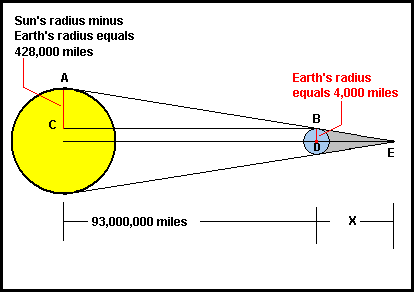Draw line 'AB' from the 'top' of the Sun to the 'top' of the Earth then draw a line from point B to point C. This forms a right triangle whose sides are 428,000 and 93,000,000 miles. (Notice that line AC is NOT 432,000 miles (the Sun's radius) because the radius of the Earth must be subtracted from this distance. The ratio of the sides of the triangle just formed is .004602150538 (428,000 ÷ 93,000,000). Now draw a line from the center of the Earth (point 'D') and extend it to the right. Extend line 'AB' until it intersects the line just drawn at point 'E'. This forms triangle 'BDE' that is similar to the triangle we previously drew. (In geometry, similar triangles have the same shape and angles but different sizes). Side 'BD' is 4,000 miles and side 'DE' has a length of 'x'. Since these two triangles ('ACB' and 'BDE') are similar, then 428,000 ÷ 93,000,000 = 4,000 ÷ x. Therefore 'x' = 869,158.878 miles. This is the length of the Earth's shadow as measured from the Center of the Earth. Subtracting the radius of the Earth (4,000 miles), the Earth's shadow measured from the Earth's surface is 865,158.878 miles. See ??? It's just that easy !!! *************************************** Puzzle #18       1/9/2000 What 2 numbers (neither of which contain any zeroes) when multiplied together equal 1,000,000,000 ? ANSWER Since 2 * 5=10 22 * 52=100 23 * 53=1,000 24 * 54=10,000 and so on, then 29 * 59=1,000,000,000 512 * 1,953,125 = 1,000,000,000 *************************************** Puzzle #17       1/3/2000 A jar contains quarters, dimes and nickels totaling \$12.90. The total number of coins in the jar is 127 and there are 3 times as many dimes as there are nickels. How many quarters, dimes and nickels are there? ANSWER The answer can be found by setting up the following 3 equations: 1) .25Q + .10D + .05N = 12.90 2) Q + D + N = 127 3) 0Q + D - 3N = 0 Solving for the 3 unknowns we obtain: 11 Quarters, 87 Dimes and 29 Nickels. *************************************** Puzzle #16       12/26/1999 Summing the powers of 2, using each power only once, any positive integer may be obtained. For example using the numbers 1, 2, 4, 8, 16, and 32 any number from 1 through 63 can be formed. For example, 9 = 8 + 1 10 = 8 + 2 11 = 8 + 2 + 1 and so on. (Remember that each power of 2 can be used only once in each sum and a sum such as 4 + 4 + 2 =10 would not be allowed). So, given these rules, how would the number 1,000,000 be obtained? ANSWER The easiest way to solve this would be to convert 1,000,000 to binary which is 11110100001001000000.Now, wherever a '1' appears, convert that to its power of 2. The first '1' equals 219, the next 218, then 217, 216, 214, 29 and 26. These numbers equal: 219 = 524,288 218 = 262,144 217 = 131,072 216 = 65,536 214 = 16,384 29     = 512 26       = 64 The sum of the above 7 numbers equals 1,000,000. *************************************** Puzzle #15       12/19/1999 The Verrazano-Narrows Bridge in New York City spans 4,260 feet between 2 towers which are each 690 feet tall. To allow for the curvature of the Earth, how much further apart must the tops of the towers be from the distance at the bottom of the towers? ANSWER An EXTREMELY precise (and involved) answer can be obtained by using trigonometry, double angle formulas, etc. However a VERY good approximation can be obtained as follows. The radius of the Earth is 4,000 miles (21,120,000 feet). Therefore, for every 21,120,000 feet of 'height' the bridge spans 4,260 feet. This makes a 4,957.75 to 1 'rise to run' ratio. (21,120,000 ÷ 4,260 = 4,957.75) So, for the final 690 feet of 'height', the bridge must span an additional (690 ÷ 4957.75) feet or .139176 feet or 1.670 inches or about 1 ⅝ inches. *************************************** Puzzle #14       12/12/1999 Returning to our Alphaland lottery (see Puzzle #13), when 3 of 6 numbers match the winning number, you win a free ticket. What is the probability of getting 3 out of the 6 winning numbers? ANSWER Using the formula from Puzzle #13, the number of ways that 3 numbers can be chosen from 6 is 20. The number of ways that 3 numbers can be chosen from the remaining 30 is 4,060. Multiplying these 2 numbers we obtain 81,200, which is the total number of ways 6 numbers can be chosen from 36 so that exactly 3 numbers will match the winning six. There are 1,947,792 possible combinations of choosing 6 numbers from 36 so the probability of having a ticket that matches 3 of the winning numbers is 81,200/1,947,792 which equals .04168822954. This means that the odds are about 1 in 24. *************************************** Puzzle #13       12/05/1999 To play the lottery in mythical Alphaland, 6 numbers are selected out of 36. In how many ways can 6 numbers be chosen from 36? (Order is NOT important, meaning 1 2 3 4 5 6 is the SAME selection as 2 3 4 5 6 1, etc.) ANSWER The formula for determining how many ways 'k' things can be selected from 'n' things is: n! ÷ (k! * (n-k)!), where the ! symbol means factorial. A factorial is determined by getting an integer, then multiplying it by that integer minus 1, and so on, until 1 is reached. For example 5! = 5 * 4 * 3 * 2 * 1, which equals 120. Returning to our problem, n = 36 and k = 6. Putting these into the formula we have: 36! ÷ (6! * (36 - 6)!) which equals 36! ÷ (6! * 30!) which simplifies to: 36 * 35 * 34 * 33 * 32 * 31 ÷ 6! which equals: 1,947,792 So there are 1,947,792 ways to pick 6 numbers from 36. *************************************** Puzzle #12       11/28/1999 What is the probability that 7 people chosen at random would have been born on a different day of the week? (That is to say, 1 born on Sunday, 1 on Monday, etc.) ANSWER The probability that 1 person would NOT be born on the same day of the week as 1 other person is six out of seven. Continuing that reasoning, with 3 people it would be 5 out of 7 and so on. So the probability that ALL seven people would be born on a different day of the week is: (6/7) x (5/7) x (4/7) x (3/7) x (2/7) x (1/7) which equals: .006119899022 or 1 in 163.4013889. *************************************** Puzzle #11       11/21/1999 Scientists define the half-life of a substance as the time in which it will lose half of its radioactivity. For example, a substance with a half-life of 10 days will have 50% of its radioactivity in 10 days, 25% in 20 days, 12.5% in 30 days and so on. A substance being tested is found to have 90% of its radioactivity after 12 days. What is its half-life in days? ANSWER The number of half-lives a substance has undergone may be indicated by the formula: Number of Half-Lives = (Elapsed Time/Half-Life) For example, if a substance has a half-life of 5 days, then after 20 days, it has undergone 4 half-lives. We will let 'n' = the number of half-lives for the next formula: Amount Remaining = (1/2n) So from the problem, we are told that the amount remaining is .9 (or 90%). Substituting into the second formula, we have: .9 = 1/2n Which can be changed to: 2n=1.1111111111111... Notice that the unknown is an exponent. When that is the case, we must solve by taking the logarithm of both sides of the equation which then becomes: n * log(2)=log(1.1111111111) n= log(1.11111111) ÷ log(2) n= .152003093 Since n represents the number of half-lives it has undergone in 12 days, this means that the half-life of the substance is 12 divided by .152003093 or 78.94576198 days. Now wasn't that easy ??? *************************************** Puzzle #10       11/14/1999 The mythical town of Alphaville had a population of 10,000 people in 1930. Since then, the population has doubled every 23 years. (For example in 1953, the population equaled 20,000; in 1976 it was 40,000; in 1999, it was 80,000 and so on). What was the population in 1980 ? ANSWER Since the Alphaville population doubles every 23 years, the population at any one time can be expressed as: POPULATION = 10,000 * 2^ (#Years ÷ 23) So, for 1980, the # of Years is 50 and the formula becomes: POPULATION = 10,000 * 2^ (50 ÷ 23) POPULATION = 10,000 * 2^ (2.173913043) 1980 POPULATION = 45,125. *************************************** Puzzle #9       11/07/1999When the Sun, Earth and Mars 'line up' (in that order), Mars is said to be in 'opposition'. Earth takes 365.25 days to orbit the Sun and Mars takes 687.0 days. How much time elapses from 1 such opposition to the next? (Astronomers call this the 'synodic period' of Mars). ANSWERThe Earth moves at .985626283 degrees per day (360 ÷ 365.25).Mars moves at .524017467 degrees per day (360 ÷ 687.0).Therefore, Earth has a net gain on Mars of .461608816 degrees per day (obtained by subtracting Mars' rate from the Earth's). So at .461608816 degrees per day, how many days would it take for the Earth to travel the 360 degrees in order to 'catch up' to Mars?360 ÷ .461608816 = 779.88 days. *************************************** Puzzle #8       10/31/1999 Ever wonder how a mountain's height is determined, especially when a tape measure cannot be brought to the peak nor can it be brought to the inside of the base of the mountain? Well, here is one way to do it (assume we are working on level ground and using a transit exactly 5 feet off of the ground). From a certain distance, the mountain peak has an angular elevation of 40 degrees. Moving precisely 1,000 feet closer to the mountain, its angular elevation is now 42 degrees. What is the height of the mountain? ANSWER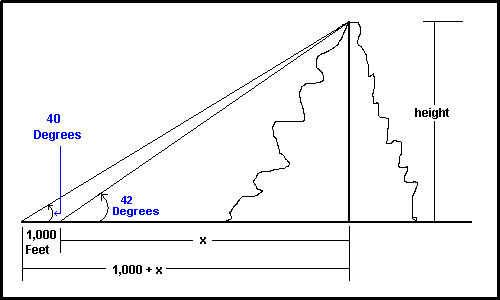The distance from the 2nd measurement will be called 'x'. The distance from the 1st measurement is therefore '1000 plus x'. We now form the 2 equations:1) tan 40 = h ÷ (1000 + x) OR h=tan 40 * (1000 + x)2) tan 42 = h ÷ x OR h=tan42 * x Where 'h' is the height of the mountain. Since h is equal to both equations then,tan 40(1000 + x) = tan 42 * xThis becomes:839.0996312 + .839099631 * x =.900404044 * x 839.0996312 = .061304413 * x Therefore x = 13,687.4 feettan 42 = h ÷ xh = .900404044 * 13,687.4Height of mountain = 12,324.2 feet plus 5 foot transit height:HEIGHT = 12,329.2 feet. *************************************** Puzzle #7       10/24/1999 A triangle has sides of 50, 70 and 80. What are its angles?ANSWER Draw a triangle diagram so that the 50 is on the left, 70 is on the right and the base is 80. Now draw a perpendicular to the base. This perpendicular will have a height 'h' another side 'x' and a hypotenuse of 50.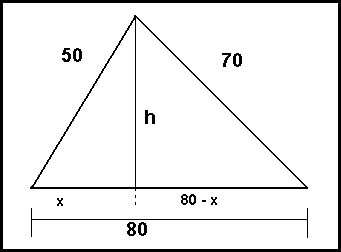From the Pythagorean Theorem, we know that h2 = 502 - x2 For the triangle on the right, h 2 = 702 - (80-x)2 Since h2 appears in both equations, we obtain a new equation of: 2,500 - x2 = 4,900 -(80-x)2 Solving this equation we find that x = 25. By substitution, we obtain h=43.30127019. From trigonometry we look up the arctangent of (h/x) = arctan(1.732050808) which equals 60 degrees exactly. The angle at the other side of the base equals arctan(43.30127019/55) or 38.2132107 degrees. Since all triangles have 180 degrees, the 3rd angle is calculated to be 81.7867893. Wow - another longwinded explanation!!! *************************************** Puzzle #6       10/17/1999 One pipe can fill a water tank in 50 minutes. Another pipe can fill the same water tank in 40 minutes. How much time would it take if both pipes were to fill the same water tank at the same time ? ANSWERThere is a formula for this type of problem but just by using logic, the answer is easily obtained. Assume the tank is 50 gallons. So the 'slow' pipe can fill it up at a rate of 1 gallon per minute. The 'fast' pipe can fill it up at a rate of 1.25 gallons per minute. Therefore, with both pipes working together, the tank is filling at a rate of 2.25 gallons per minute. Since it is a 50 gallon tank, then it will fill up in (50 ÷ 2.25) minutes or 22 minutes and 13⅓ seconds. If you want to use the formula, it is (Rate1 x Rate2) ÷ (Rate1 + Rate2) = Time. *************************************** Puzzle #5       10/10/1999 An employee is to be rewarded with a \$250 bonus AFTER TAXES have been deducted. If the federal tax is 15%, the state tax is 10% and the Social Security is 7.5 % what must the GROSS PAY be in order for the net pay to be \$250 ? ANSWER: Adding the 3 tax rates together gives us a total tax rate of 32.5%. 1)Tax = Gross Pay * .3252)Gross Pay - Tax = Net Pay Substituting Equation 1 into 2 produces:Gross Pay -.325*Gross Pay =Net Pay. Since we are looking for \$250 Net Paythen Gross Pay -.325*Gross Pay = \$250 .675 * Gross Pay = \$250Gross Pay = 370.37 *************************************** Puzzle #4       10/03/1999Twelve is the smallest positive integer that can be divided by the integers 1, 2, 3 and 4 with no remainder. What is the smallest positive integer that can be divided by 1, 2, 3, 4, 5, 6, 7, 8, 9, and 10 without any remainder? ANSWER: This number would have to have as its factors 1, 2, 3, 4, 5, etc. Since any number has 1 as a factor, we don't need it. We will need the 2 and 3. When we get to 4, we already have 2 as a factor so we just need another 2. 5 is prime so we definitely need that. We already have 6 as a factor because 2 x 3 = 6 so we don't need to add the '6'. 7 is prime and so we will need that. We do not need 8 as a factor because it is 2x2x2 and since we already have a '4', we just need another 2. We already have a 3, so we just need another 3 to make a '9'. We do not need the 10 because 2x5 = 10. Therefore our number is:2 x 3 x 2 x 5 x 7 x 2 x 3 = 2,520. *************************************** Puzzle #3       9/26/1999This one is a 'classic' (and even Marilyn Vos Savant got it wrong - no kidding).If a chicken and a half can lay an egg and a half in a week and a half, how many eggs would one chicken lay in a month and a half (6 weeks) ?ANSWER: The common mistake is to reduce all three quantities to 1 1 chicken lays 1 egg in 1 week. However, if you think carefully about it, this is not the case. For example, imagine you have 8 painters in your 8 room house and they finish in 8 hours. Would this mean 1 painter could paint 1 room in 1 hour? Heck no! One painter can paint one room in EIGHT HOURS. Returning to the puzzle, we then can say that one chicken lays one egg in a WEEK AND A HALF.Therefore, 1 chicken could lay FOUR EGGS in a month and a half. *************************************** Puzzle #2       9/17/1999 A square piece of wood measures 2 feet on each side.If it is cut into a regular octagon (8 sided figure, all sides and angles equal), what does each of the 8 sides measure? ANSWER:Using Geometry: Construct a square in the 'upper left' side of the square. Each of the sides of this square would be 1 foot because the piece of wood is 2 feet square. Using the Pythagorean Theorem, the diagonal of this smaller square would be the square root of 2 or 1.414213562. Now if we draw a line at the upper left to represent one of the sides of the octagon, it would 'slice' the diagonal at precisely the 1 foot mark with the remaining segment of the diagonal equal to .414213562 feet.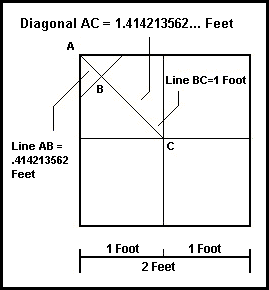This segment is precisely one half the length of 1 of the sides of the octagon because it is part of a 90-45-45 triangle. Therefore, each of the octagon's sides would equal .828427124 feet or 9.941125497 inches. Using Trigonometry:From the center of the square, draw a perpendicular bisector to one of the sides of the octagon. The height of this bisector is 1 foot. Since each side of the octagon subtends a 45 degree angle with the center, then the angle subtended by the perpendicular bisector and a line to the end of 1 side would be 22.5 degrees. The 'half-side' length would equal tan 22.5 degrees times 1 foot which equals .414213562 feet and the full-side length is double this or .828427124 feet or 9.941125497 inches.WOW - were those explanations lengthy or what ???????????? *************************************** Puzzle #1       9/12/1999This is not as simple as it appears at first glance.A car travels at 60 miles per hour over a certain distance. The car makes the return trip over the exact same distance at 30 miles per hour. What is the average speed of the car? ANSWER: Let us suppose that the 'one-way' distance is 60 miles. So, on the 'outbound' journey, the car would take 1 hour.On the return trip the car would take 2 hours.So, the 'round-trip' is 120 miles and the car would take 3 hours to make the trip. 120 miles divided by 3 hours = FORTY MILES PER HOUR. _____________________ Return To Home Page Copyright © 1999 -     1728 Software Systems